Test: Control System - 3

# Test: Control System - 3 - Electrical Engineering (EE)

Test Description

## 25 Questions MCQ Test GATE Electrical Engineering (EE) 2023 Mock Test Series - Test: Control System - 3

Test: Control System - 3 for Electrical Engineering (EE) 2023 is part of GATE Electrical Engineering (EE) 2023 Mock Test Series preparation. The Test: Control System - 3 questions and answers have been prepared according to the Electrical Engineering (EE) exam syllabus.The Test: Control System - 3 MCQs are made for Electrical Engineering (EE) 2023 Exam. Find important definitions, questions, notes, meanings, examples, exercises, MCQs and online tests for Test: Control System - 3 below.
Solutions of Test: Control System - 3 questions in English are available as part of our GATE Electrical Engineering (EE) 2023 Mock Test Series for Electrical Engineering (EE) & Test: Control System - 3 solutions in Hindi for GATE Electrical Engineering (EE) 2023 Mock Test Series course. Download more important topics, notes, lectures and mock test series for Electrical Engineering (EE) Exam by signing up for free. Attempt Test: Control System - 3 | 25 questions in 75 minutes | Mock test for Electrical Engineering (EE) preparation | Free important questions MCQ to study GATE Electrical Engineering (EE) 2023 Mock Test Series for Electrical Engineering (EE) Exam | Download free PDF with solutions
 1 Crore+ students have signed up on EduRev. Have you?
Test: Control System - 3 - Question 1

### The OLTF of closed loop system is given by the following Transfer function.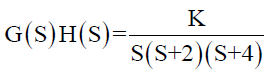The maximum value of K for which the system just become unstable is-

Detailed Solution for Test: Control System - 3 - Question 1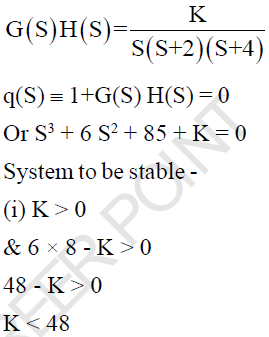Test: Control System - 3 - Question 2

### Which principle specifies the relationship between enclosure of poles & zeros by s-plane contour and the encirclement of origin by q(s) plane contour?

Test: Control System - 3 - Question 3

### The control system shown in the given fig has an internal rate Feedback loop. The closed-loop system for open and closed condition of the switch will be respectively-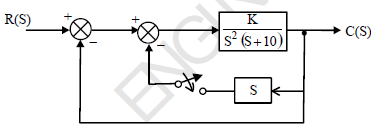Detailed Solution for Test: Control System - 3 - Question 3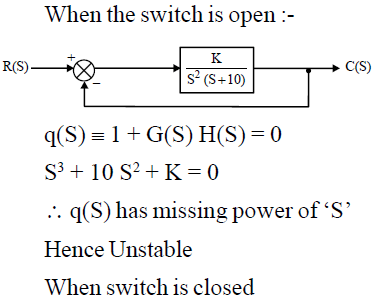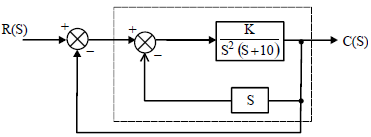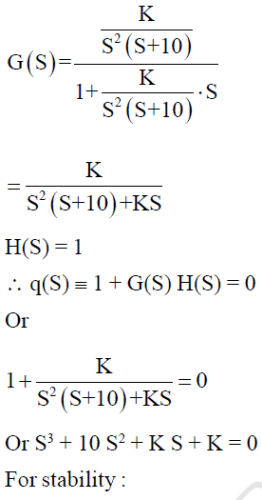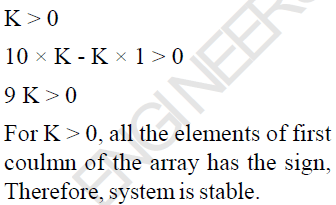*Answer can only contain numeric values
Test: Control System - 3 - Question 4

An under damped second order  system having Transfer function of the form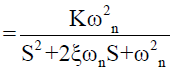has a frequency response shown below, The system gain ‘K’ is...?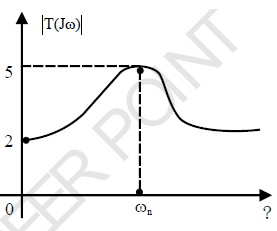Detailed Solution for Test: Control System - 3 - Question 4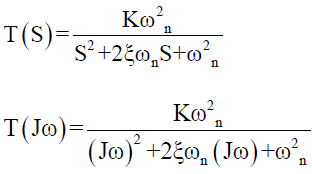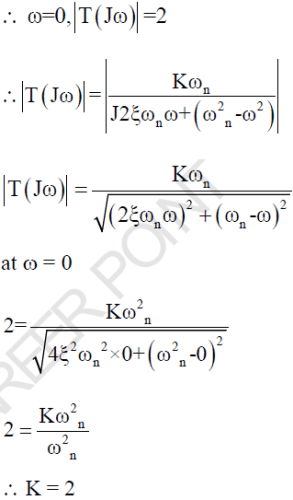Test: Control System - 3 - Question 5

The OLTF of -ve UFB control system is given by-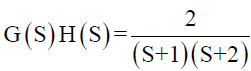The gain margin (in dB) is given by-

Detailed Solution for Test: Control System - 3 - Question 5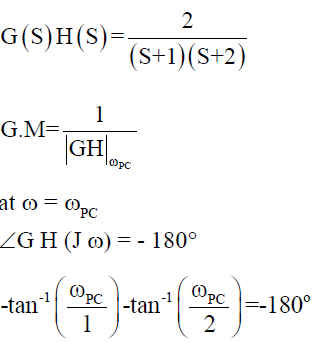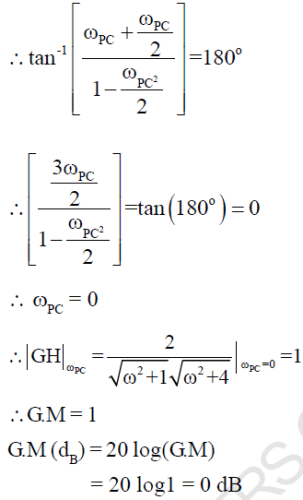Test: Control System - 3 - Question 6

A unity Feed back system has forword loop gain T.F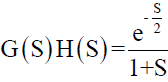. The cross over frequency (ωPC) is given by -

Detailed Solution for Test: Control System - 3 - Question 6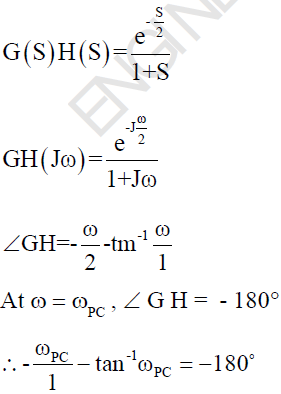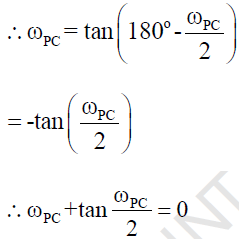Test: Control System - 3 - Question 7

The Magnitude Bode Plot of a certain system is shown below.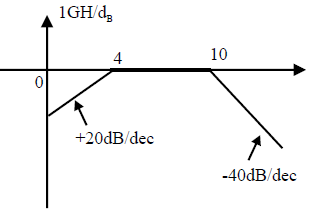What will be its Transfer function..?

Detailed Solution for Test: Control System - 3 - Question 7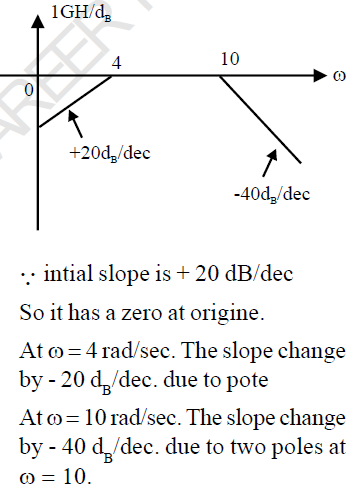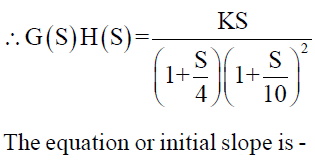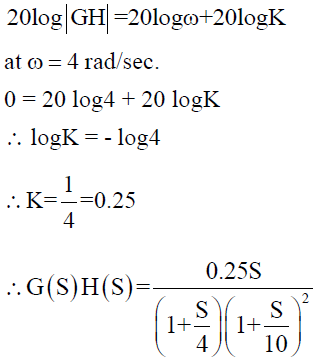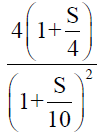Test: Control System - 3 - Question 8

The T.F. of control system is given by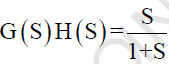. The polar plot of the system lies in-

Detailed Solution for Test: Control System - 3 - Question 8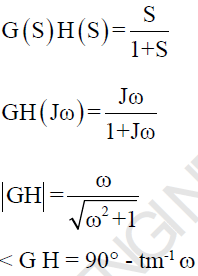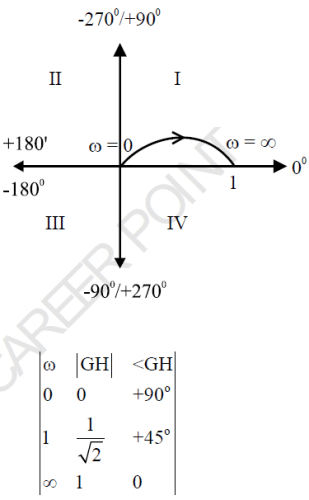Test: Control System - 3 - Question 9

Which terminology deals with the excitation or stimulus applied to the system from an external source for the generation of an output?

Detailed Solution for Test: Control System - 3 - Question 9

For the output of the system, mainly the input and state of the system are required and the excitation or stimulus applied to the system from an external source for the generation of the output is called input signal.

Test: Control System - 3 - Question 10

The response C(t) of a system to an input r(t) is given by the following differential equation: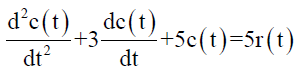The Transfer function of the system is given by-

Detailed Solution for Test: Control System - 3 - Question 10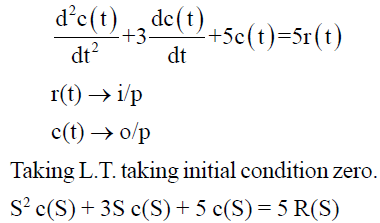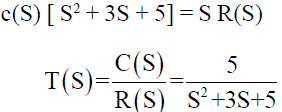Test: Control System - 3 - Question 11

A second order system is defined by the following differential equation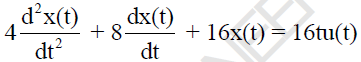The damping ratio and natural prog. For this system is respectively.

Detailed Solution for Test: Control System - 3 - Question 11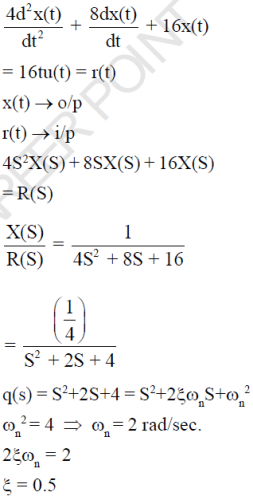Test: Control System - 3 - Question 12

For the given system the magnitude of CLTF sensitivity w.r.t OLTF at  frequency ω = 1 rad/sec is ?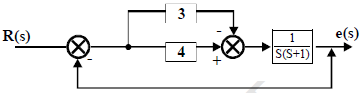Detailed Solution for Test: Control System - 3 - Question 12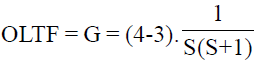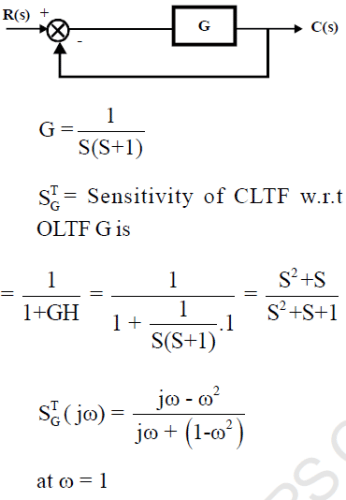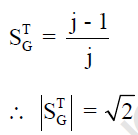Test: Control System - 3 - Question 13

Find the impulse response of the system having transfer function each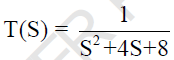Detailed Solution for Test: Control System - 3 - Question 13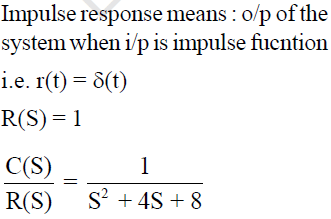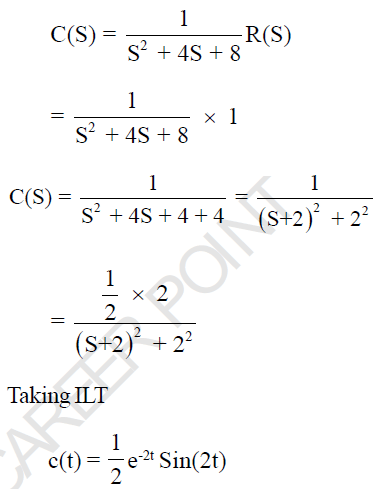Test: Control System - 3 - Question 14

A closed loop control system has G(S) = 100/S2 and H(S) = (S+1) The steady state output for a unit step
input is…?

(Important - Enter only the numerical value in the answer)

Detailed Solution for Test: Control System - 3 - Question 14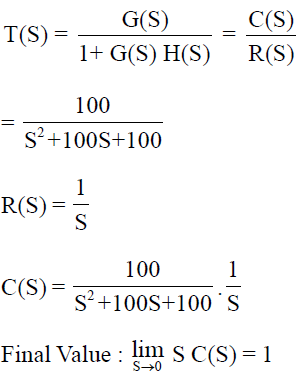Test: Control System - 3 - Question 15

A linear time invariant system initially at rest, when subjected to unit step input gives a response y(t) = te-t, t > 0 The corresponding transfer function is

Detailed Solution for Test: Control System - 3 - Question 15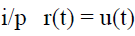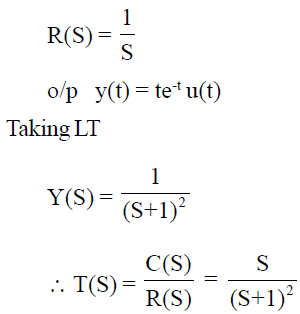Test: Control System - 3 - Question 16

The responsse of a system is shown by the given wave-form. The correct root location in the S- Plane is ..?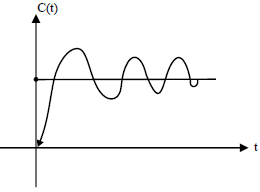Detailed Solution for Test: Control System - 3 - Question 16

Given fig is response of the system which is under-damped

i.e. 0<ξ<1

For under-damped system, Poles are Comlex-Conjugate and lies on left half of S-Plane.

*Answer can only contain numeric values
Test: Control System - 3 - Question 17

Consider a causal L T I system characterized by the Differential equations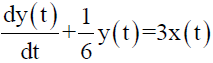The steady state o/p of the system to input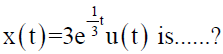(Important - Enter only the numerical value in the answer

Detailed Solution for Test: Control System - 3 - Question 17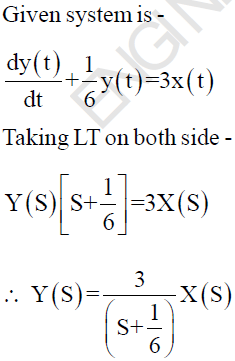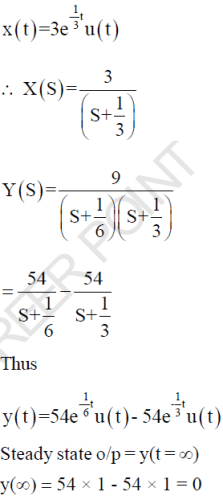Test: Control System - 3 - Question 18

The system shown in the given fig. is subjected to a unit rump input.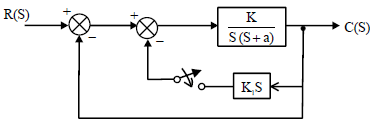On closing the switches

Detailed Solution for Test: Control System - 3 - Question 18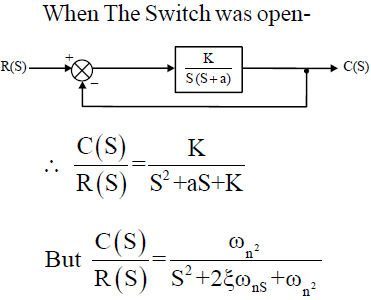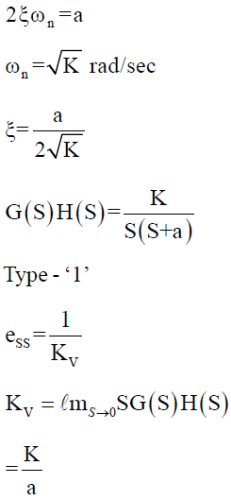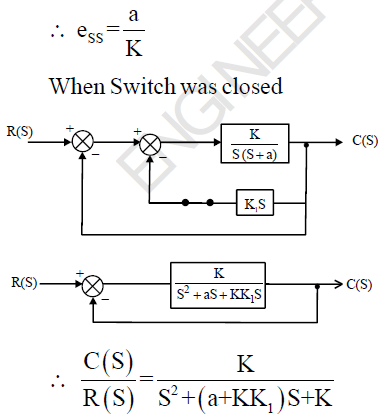Test: Control System - 3 - Question 19

The impulse response of an initially relaxed linear system is e-2t , t > 0. To produce a response of t e- 2t , t >0, The input must be equal to -

Detailed Solution for Test: Control System - 3 - Question 19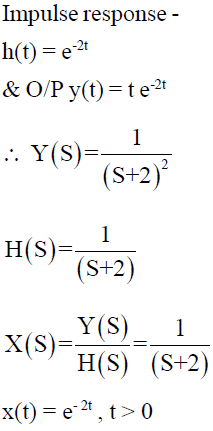Test: Control System - 3 - Question 20

A control system is discribed by the following equation -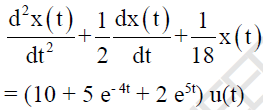The Natural time constants of the system are-

Detailed Solution for Test: Control System - 3 - Question 20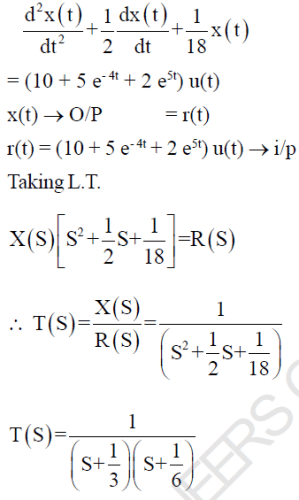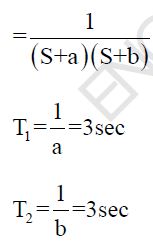Test: Control System - 3 - Question 21

The root location for a second order system is shown, the dumping ratio and natural freq. of system is respectively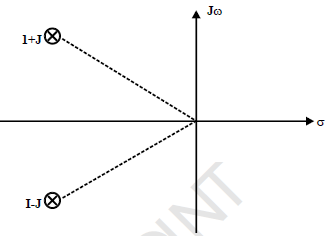Detailed Solution for Test: Control System - 3 - Question 21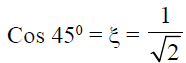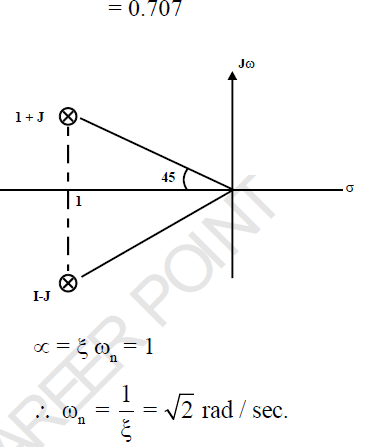Test: Control System - 3 - Question 22

Consider UFB system with forward path T.F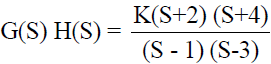The range of K for which system is stable

Detailed Solution for Test: Control System - 3 - Question 22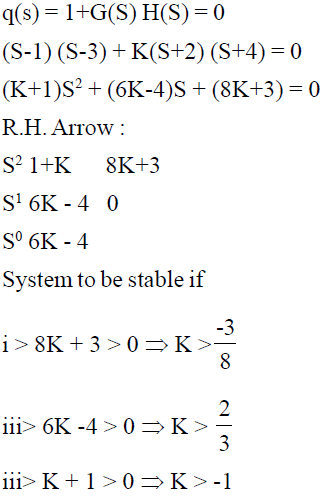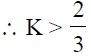*Answer can only contain numeric values
Test: Control System - 3 - Question 23

An open loop control system results in a response of

(10e-2t - 3e-t) u(t)

For an unit impulse input. The D.C. gain of control system is….....?

(Important - Enter only the numerical value in the answer)

Detailed Solution for Test: Control System - 3 - Question 23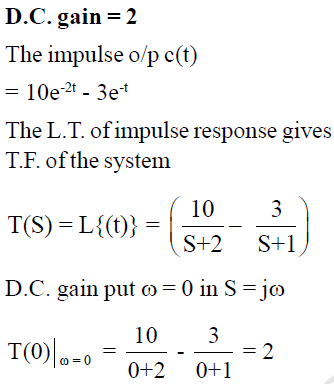Test: Control System - 3 - Question 24

For the given system the value of  K and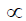so that the system oscillates at freq. ω = 1 rad/sec.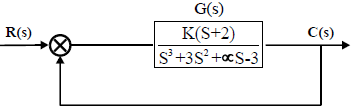(Important - Enter only the numerical value in the answer)

Detailed Solution for Test: Control System - 3 - Question 24

K = 3 &= -2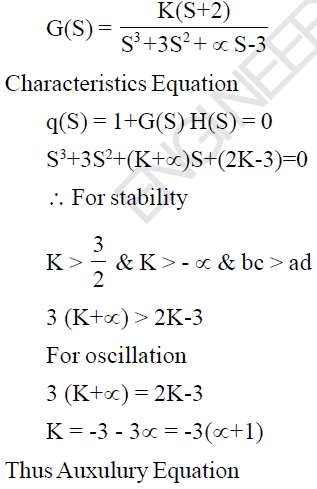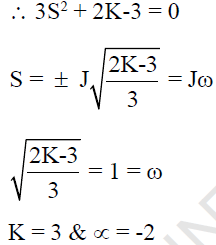*Answer can only contain numeric values
Test: Control System - 3 - Question 25

A system with derivative controller is given as below. The value of KD for which the damping ratio becomes 0.8 is ?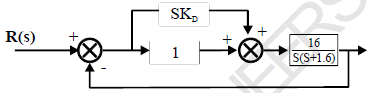(Important - Enter only the numerical value in the answer)

Detailed Solution for Test: Control System - 3 - Question 25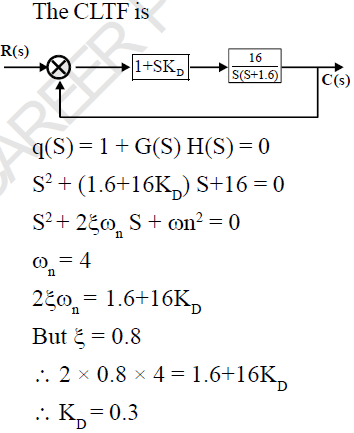## GATE Electrical Engineering (EE) 2023 Mock Test Series

22 docs|284 tests
Information about Test: Control System - 3 Page
In this test you can find the Exam questions for Test: Control System - 3 solved & explained in the simplest way possible. Besides giving Questions and answers for Test: Control System - 3, EduRev gives you an ample number of Online tests for practice

## GATE Electrical Engineering (EE) 2023 Mock Test Series

22 docs|284 tests(Scan QR code)